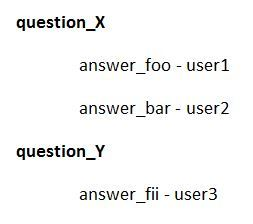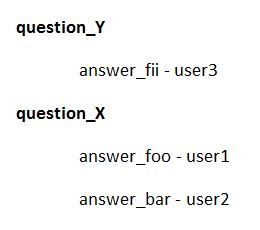# Enum.group_by doesn´t keep sort order

``````x = [%{position: 1, question: "Question2"}, %{position: 2, question: "Question1"}, %{position: 2, question: "Question1"}]
y = Enum.group_by(x,&(&1.question))
``````

gives me

``````%{"Question1" => [%{position: 2, question: "Question1"},%{position: 2, question: "Question1"}], "Question2" => [%{position: 1, question: "Question2"}]}
``````

I need the sort order to be the position. So i get this:
edit:

``````%{"Question2" => [%{position: 1, question: "Question2"}],"Question1" => [%{position: 2, question: "Question1"},%{position: 2, question: "Question1"}]}
``````

How can i achieve that?

thanks

1 Like

You can sort afterwards I guess?

1 Like

The expected result you showed is different even if you do sort the results. In the input you have one element `%{position: 1}` and in your expected results there are two elements.

`Enum.group_by` keeps the input order on the lists it creates for each group. The map keys are not sorted because maps are not an ordered data structure. The order is an implementation detail of the runtime.

EDIT: If you need sort the lists, you can either sort the input list because `Enum.group_by` keeps that order. Or you can sort each individual list in the result by doing this: `Enum.into(result, %{}, fn {key, list} -> {key, Enum.sort(list)} end)`.

2 Likes

Thanks. But i don´t really get it. Where do you see only one element? Every element contains position and question.

I don´t see how `Enum.into...` can achieve the expected result. I don´t want to sort the lists inside the elements but rather the result as a whole. For example, if i have a question with a position 1 like this:
`%{position: 1, question: "Question2"}` i need the the Question2 to be on top of every other Question with a lower position after the questions have been grouped.

1 Like

Your first example: `%{"Question1" => [%{position: 2, question: "Question1"},%{position: 2, question: "Question1"}], "Question2" => [%{position: 1, question: "Question2"}]}`

Your second example: `%{"Question2" => [%{position: 1, question: "Question2"},%{position: 1, question: "Question2}], "Question1" => [%{position: 2, question: "Question1"}]}`

The first example has two maps with `position: 2`, your second example has only map with `position: 2`. Sorting won’t change that :).

You can’t sort the results as a whole because maps are not ordered. If you want change the order of the keys you need to use a list and sort the list.

It’s hard to see exactly what you want because the examples you showed will not be correct even after sorting.

1 Like

Oh, sorry. I´ve edited the second example. But i see know, that map doesn´t seem to offer what i´m looking for.

This is the table i have

What i have at the moment:What i need is the questions sorted by the position in the table row.I´d like to group the questions so answers from users can be compared by question.

1 Like

I think something like this should work if all the positions are the same for a group:

``````Enum.sort_by(result, fn {_key, value} -> hd(value).position end)
``````

`hd(value).position` will extract the first position from the grouped list.

We need to transform the map to a list here to maintain the sorted order and `Enum.sort_by` does that for us.

2 Likes

Thank you very much for your time and help!

1 Like